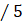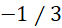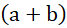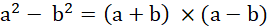or# RD Sharma Class 9 and Chapter Factorization Of Algebraic Expressions Maths Solutions

## Maths Class 9 and Chapter Factorization Of Algebraic Expressions RD Sharma SolutionsFilter
Filters :
CLASSES
•
•
•
•
•
•
•
CHAPTERS
•
• 15 More

# RD Sharma Class 9 Maths Chapter 5 Factorization of Algebraic Expressions

In class 9 RD Sharma’s textbook, one can find this unit called ‘Factorisation of Algebraic Expressions’ that seems to be the next level unit of the ‘Algebraic Identities’ that was previously discussed in the book. The previous unit talked about the derivations of algebraic expressions and the list of standard algebraic expressions that would be very useful during the process of factorisation. And this chapter would be all about factorisation. The combined knowledge of these two would turn out to be a great tool when solving complex algebraic equations.

Talking of algebra, all the parts of it are somewhat interrelated and hence the basic concepts such as the algebraic expressions, identities, factorisation, etc. have to be learnt pretty well. Sound knowledge of these units is to be carried forward through the further classes and hence it is important to ensure that the basic concepts are mastered well.  Learning a chapter in the right way requires the usage of appropriate study material.  For the students in India studying under the CBSE board of education, the RD Sharma books are said to be a boon!

The RD Sharma books are quite popular among the student crowd in India for many years now. Dr. R.D. Sharma, a maths professor, has over 30 years of experience in authoring maths books now. Over these years, through his captivating skills, Dr R.D. Sharma has made many students’ lives easier through his mathematics books. The speciality of the RD Sharma maths books is the simplicity and at the same time the depth with which even the complex concepts are presented. Hence, the RD Sharma books have become the go-to medium for the students. Also, the books come in line with the latest CBSE syllabus, thus making them the obvious choice for the students.

The step-by-step methodology used to solve the problems and the interesting problems put across in the form of exercises in these textbooks form the basic essential for the students to face the exams confidently. Not just that, the RD Sharma books are found to be helpful for the ones who would want to crack India’s toughest entrance exams like JEE, too!

Generally, a subject like maths requires the students to procure a good solution guide for the reference textbook used in order to make things easy. One of the premium online tutorial platforms where the students can find the solutions to the textbook in the form of videos is the doubtnut.com. One can find all the problems in RD Sharma’s textbook solved exercise-wise. RD Sharma Class 9 Solutions Chapter 5 Factorization of Algebraic Expressions found on the doubtnut.com can be accessed easily and used for reference as and when required.

Factorization of Algebraic Expressions: Class 9 Maths RD Sharma Solutions

Talking of the meaning of a factor, it is nothing, but the quantity that when multiplied with other quantities result in an expression. Each ‘multiple’ that form a part of an algebraic expression can be called a factor. For example, let’s take the number 6. The number 6 can be expressed as 3*6. Hence, we can say that the factors of 6 are 3 and 2. Similarly, the algebraic expressions can also be expressed in the smallest terms by means of factorisation. Thus, we can tell that factorisation is nothing but expressing a number or an algebraic expression in terms of its smallest terms.

Coming to the algebraic expressions, they are found to be in the form of monomials, binomials and polynomials. Monomials are the expressions that have only one term, binomials have two terms, trinomials are the expressions having three terms, and polynomial has a greater number of terms. Solving binomials and trinomials are going to be quite an easy task but not the polynomials. Almost all equations except the monomials do require to be factorised and the level of factorisation depends on the complexity of the expression.

Let’s see how the algebraic expressions can be factorised. Take an example of a binomial equation, 5x2+x = 0. This expression can be factorised into the form of, x(5x+1) = 0.

Now, we can say that, x = 0 (or) 5x+1 = 0.

Taking 5x + 1 = 0, 5x = -1, x = -1∕5Thus, it can be concluded that the process of factorisation the expression helped in finding the value of the variable ‘x’. It can be seen that in the above example, the factorisation is called ‘factorisation of the algebraic expressions by using the common terms’. Sometimes, another process called ‘regrouping the terms’ could be essential before factorisation.

Similarly, the factorisation can be done by the process of splitting the middle term. This method is commonly used on the trinomial expressions. Taking an example for the same, 3x2- 2x-1 = 0

3x2 - 3x + x - 1 = 0

3x (x - 1) + 1 (x – 1) = 0

(3x + 1) (x – 1) = 0

x = 1, x = -1∕3It can be noted that the trinomial equation is now solved by the process of factorisation by spitting the middle terms.

Factorisation of the algebraic expressions by using the standard identities is another way of factorising the expressions. Chapter 4 in RD Sharma’s book has covered everything about the standard identities. Most of the students by now would be aware of the standard identities that would play a great role in the factorisation of the polynomial. And some of these important identities include:

a+b2 = a2 + 2ab + b2

a+b2 = a2 + 2ab + b2

a2- b2=a+b ×(a-b)(a + b)= a3 + b3 + 3ab (a + b)

(a - b)= a3 - b3 - 3ab (a - b)

a3 + b3 = (a + b) (a2 + b2 – ab)

a3 – b3 = (a – b) (a2 + b2 – ab)

(x + y +z)2=x2 +y2 + z2+2xy+2yz+2x

All these techniques of the factorisation are included in this unit and the learners would also see the exercise questions appearing based on these concepts. These techniques have to be learnt well and hence there requires a lot of practise from the students. In order to face the questions asked in the examinations smoothly, the students must be able to picturise the solution technique as soon as they spot the questions and such must be their knowledge level in this unit. Doubtnut provides the much-required guidance and support by providing the solutions worked out in videos. The students can see that an expert teacher takes them through the complex concepts in an orderly manner.

## Topics and Subtopics of RD Sharma Class 9 Maths Chapter 5 Factorization of Algebraic Expressions

Now that there are so many things to remember and learn in this chapter, RD Sharma Class 9 Maths Chapter 5 Factorization of Algebraic Expressions, it is essential to first be aware of the chapter content in a nutshell. This is when knowing the topics and subtopics in the chapter becomes important. This way, it gets easier for learners to plan and study accordingly. Now looking at the topics and subtopics of the chapter based on which the RD Sharma textbook solutions are presented at doubtnut.com.

### Introduction to Factorisation

The meaning, the terms and other important basics are introduced.

### Factorisation by common terms

One can spot a lot of problems appearing in RD Sharma’s textbook are based on the concept of the common terms.

### Factorisation by factor method

The problems from here mainly involves the process of splitting the middle term in order to arrive at the solutions.

### Factorisation by using the identities

The process factorisation by using identities is an important concept based on which one can find the majority of the exercise questions being asked.

Looking at the content, it can be concluded that this chapter forms a very important one in the study of algebra. One can expect a lot of questions coming from this unit on the exams, both the CBSE exams as well as the entrance exams. Hence it is important to practise more and more from the textbook. All solutions are found on the doubtnut.com, and any doubts can be cleared in no time by a simple process of posting a picture of the doubt question. This platform just serves like a live virtual guide who conveys the proper way of approaching all problems and also clears any sort of doubts that could occur at any point of time among the students. This, in turn, boosts a lot of confidence in the students to face the examinations. Doubtnut has now grabbed the attention of thousands of students from all over India and they find it really helpful during the exam preparations. One can benefit from over 50 Lakh videos that include both textbook solutions and IIT JEE preparations that are found on the doubtnut.com!